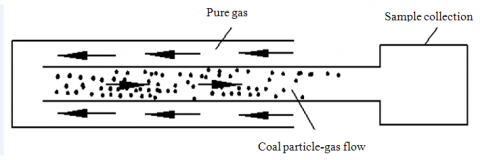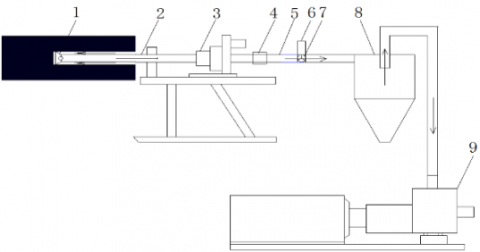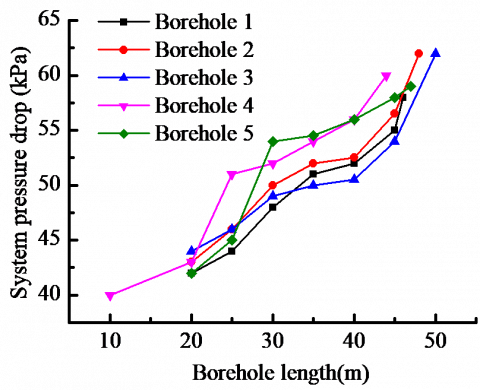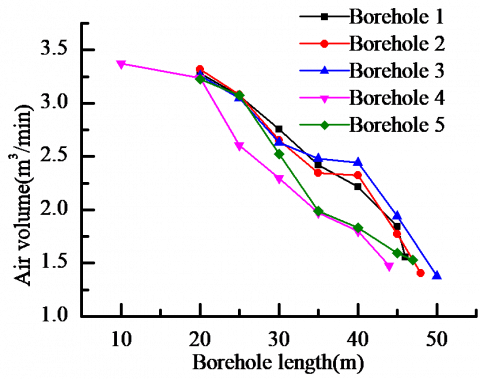# Application of Sampling Method Based on Negative Pressure Pneumatic Conveying in Soft Coal Seam

Application of Sampling Method Based on Negative Pressure Pneumatic Conveying in Soft Coal Seam

Hongtu Zhang Jianping Wei Yungang Wang* Zhihui Wen Banghua Yao

State Key Laboratory Cultivation Base for Gas Geology and Gas Control, Henan Polytechnic University, Jiaozuo 454000, Henan, P. R. China

School of Safety Science and Engineering, Henan Polytechnic University, Jiaozuo 454000, Henan, P. R. China

Coal Production Safety Collaborative Innovation Center in Henan Province, Jiaozuo 454000, Henan, P. R. China

Corresponding Author Email:
wyg@hpu.edu.cn
Page:
473-478
|
DOI:
https://doi.org/10.18280/ijht.340317
|
Accepted:
|
Published:
30 September 2016
| Citation

OPEN ACCESS

Abstract:

Accurately determining coalbed gas content is difficult using the existing sampling methods in China. In this paper, the drawbacks of the sampling method based on positive pressure pneumatic conveying were analyzed. The sampling method based on negative pressure pneumatic conveying was presented aiming at increasing the coal sampling purity. Furthermore, the two key parameters of power device used during the process were determined using the multi-phase theory. Then, on-site tests were carried out to test the feasibility of the application of the sampling method based on negative pressure pneumatic in the soft coal seam of the Xin’an Coal mine by the self-developed sampling device. The result showed that the effect of drilling pipe rotation on the coal along the hole and poor stability of the hole in the soft coal seam led to low coal sampling purity with positive pressure pneumatic in the sampling method The sampling method based on negative pressure pneumatic avoided the drawback of the sampling method based on positive pressure with higher coal sampling purity and shorter time consumption. The coal sampling with a length of 50m hole can be achieved within 0.5 min by the negative pressure pneumatic conveying sampling.

Keywords:

Drill pipe inner diameter, Drilling velocity, Negative pressure pneumatic conveying, Particle breakage ratio.

1. Introduction

Gas content is a key basic index for controlling gas disasters in coal mine. A Chinese standard, the direct method of determination of coalbed gas content in a mine has been issued in 2009 . In the standard, determining coal mine gas content includes three parts; i.e., sampling process, on-site coal sample desorption, and coal sample desorption in lab. These correspond to the three main parts of gas content; i.e., gas loss amount, on-site gas desorption amount, and residual desorption amount in lab. In practice, it is difficult to determine an accurate value for the gas loss amount due to the drawbacks of low sample purity or long exposure time of the existing sampling methods; i.e., the core tube sampling method and sampling method based on positive pressure pneumatic conveying (SMPPPC). According to , the core tube sampling method or other sampling methods are recommended to sample from the designed place within five minutes. However, this is a time consuming and complex operation process resulting in bad sampling sealing, thus yielding a lower gas content. Soft coal seams are a common occurrence in China, and the core tube sampling method is difficult to apply due to the poor stability of the borehole [2-4]. The latter method has been widely used in many coal mines due to its short time consumption and ease of operation, but the sample purity achieved by this method is poor. So, the above mentioned methods are unable to achieve the sample from the designed place with high purity within five minutes. In recent years, in order to improve the sample purity, some new sampling methods have been proposed [5-7]. In the new methods, the sampling method based on the negative pressure pneumatic conveying (SMNPPC), with a simple sampling device, is easy to operate and has a high sample purity obtained with a shorter time-consumption has been presented .

In this study, the drawbacks of SMPPPC has been presented. Then the key parameters of SMNPPC were calculated. The SMNPPC theory and the sample device were also presented and the feasibility of this method was validated by the on-site tests. The results provide a base for the design of SMNPPC.

2. The Drawbacks of Smpppc

2.1 Drawbacks of sampling with triangular drilling rod

The common  drilling rods in the process of determining the gas content in the coal seam are classified into three kinds for their shapes of the cross-section; i.e., triangular drilling rod, circular drilling rod and the spiral drill rod. In practice, the real mass of coal particles is 3～6 times of that in theory , meaning that a larger fluid area is needed to carry out the drilling cuttings. The spiral drilling rod has been widely used in soft coal seam for the drilling rod carrying the drilling cuttings by the force acting on them, with the blades in the rod and the drag force induced by the fluid. The triangular drilling rod has a larger fluid area than the circular drillingrod at a certain rod diameter, and the triangular drilling rods are also widely used in the soft coal seams in China. Figure 1 shows the drilling cuttings conveying process with a triangular drill rod.

## figure_1.png1-driling cuttings lift area, 2-spiral drill rod, 3-drilling cuttings descending area, 4- borehole, 5-drilling cuttings deposit area

Figure 1. Drilling cuttings conveying process with triangular drill rod

The Figure 1 shows the details about the drilling cuttings conveying process with a triangular drill rod. In the process, for the gravity , the drill rod was erratic to the bottom part of the borehole . The drilling cuttings distribution area was divided into three parts; i.e., the drilling cuttings lift area, descending area and the deposit area. The drill rod rotates at a certain speed and plays an important role in carrying the drilling cuttings. With the drilling forward, drilling cuttings are exposed to the air when it is released from the coal wall and the exposing time is different with the length of borehole. The particle pathline of drilling cuttings from the pit area is spiral and circulates from the lift area, descending area, deposit area, and lift area for the drill rod rotation. Therefore, the drilling cuttings with short exposure time were mixed with those with long exposure time, and the time was difficult to determine. The keys in determining gas loss content accurately are to obtain samples with similar time exposure and determine the accurate exposure time. However, both the above mentioned factors are difficult to obtain by this method.

2.2 Drawbacks of sampling with spiral drilling rod

Figure 2 shows the scheme diagram of sampling with a spiral drilling rod. With the drilling moving forward and the drilling rod rotating at a certain speed, there are two kinds of forces acting on the drilling cuttings. One is the force induced by the blades on the body of rod, which are rotating with the rod body and pushing the drilling cuttings out. The other force is the compressed air acting on the drilling cuttings.

## figure_2.png1-pit, 2-borehole, 3-blade, 4- drilling cuttings,  L1-fresh drilling cuttings(with low exposure time),  L2-deposite fresh drilling cuttings (with long exposure time)

Figure 2. Coal particle conveying process with spiral drill pipe

Figure 2 shows the coal particle conveying process with a spiral drill pipe. The whole borehole length was divided into two sections; one with a shorter exposure time with a length L1, and the other one with longer exposure time with a length L2. In order to obtain the coal samples from the portion L1, the coal particles in portion L2 should first be collected. One problem is that it is difficult to determine whether the coal samples are from the L1 or not. The other problem is the determination of accurate time consumption of the sampling process.

At the same time, during the sampling process, the temperature of coal particles was heated induced by the friction and collision of coal practiceparticle, particle-blades. However, some previous studies revealed that the higher the temperature, the faster the gas desorption velocity [10-12]. While the loss of gas content was usually measured by some empirical formulas that are obtained under normal atmospheric temperature. So the temperature increase induced by friction and collision enlarge the deviation of determination of gas loss content.

3. Theory of Smnppc

SMNPPC has obtained wide attention from researchers, especially in the Chinese coal mining industry. The sampling method is different from the existing sampling method based on the positive pressure pneumatic conveying. In SMNPPC, pure air enters the borehole through the annular space into the pit, while at the same time, coal particles seperate from the coal wall. The coal particle-gas two phase flows through the fluid hole into the drilling rod inner section to the sampling collection device. Compared to existing methods, this process has three advantages. The sample flows through the drilling rod inner section and there is no coal particle distribution in the borehole surface mixed into it, thus leading to high sample purity. Furthermore, the drilling rod ration reduces the risk of coal particle deposit in the borehole and shortens the coal particle movement time. Lastly, the whole process is easy to operate.

4. Parameters for The Smnppc

The power source of the SMNPPC is supplied by the vacuum pump. Working pressure and air flow rate are the two main performance parameters. The energy of coal particle–gas flow is supplied by the vacuum pump and in order to make sure the sampling process is successful, the performance parameters of the vacuum should meet the demands.

4.1 Determination of vacuum pump working pressure

The vacuum pump working pressure is equal to the whole pressure drop induced by the gas/gas-solid flow. As for the SMNPPC, the pressure drop can be divided into three parts; i.e., the pure gas flow pressure drop $\Delta P_{\mathrm{f}}$ , gas-solid flow pressure drop $\Delta P_{\mathrm{mf}}$ , and the sample collection pressure drop $\Delta P_{\mathrm{s}}$ . Figure 3 shows the scheme diagram of the pressure drop component.

## figure_3.pngFigure 3. Scheme diagram of pressure drop component

4.1.1 Pure gas flow pressure drop $\Delta P_{\mathrm{f}}$

The pure gas is exhausted by the vacuum pump, flowing through the annular space between the borehole and external drilling rod. The boreholes aiming at determining the gas content are always along the coal seam occurrence dip angle. The horizontally inclined coal seamdip slope angle is lower than 8 degrees, and the main pressure drop is induced by the friction. The pure gas flow pressure drop $\Delta P_{\mathrm{f}}$  is given as follows:

$\Delta P_{\mathrm{f}}=\lambda_{\mathrm{g}} \rho \frac{L v^{2}}{2 D^{\prime}}$(1)

where , λg is the friction coefficient λg=0.0125+0.0011/D; ρ is the gas density kg/m3; L is the borehole length m; Dis equivalent diameter of the annular space between the borehole and external drilling rod m; vg is the gas velocity m/s.

4.1.2 Gas-solid flow pressure drop $\Delta P_{\mathrm{mf}}$

According to the additional pressure drop theory , $\Delta P_{\mathrm{mf}}$  equals the whole pressure drop induced by the gas flow and particle flow, ΔPg and ΔPp, respectively. $\Delta P_{\mathrm{mf}}$  includes four parts, acceleration pressure drop, suspending and lift pressure drop, steady flow friction pressure drop and the local pressure drop. In a general pneumatic conveying process, the acceleration pressure drop is small and can be disregarded. Because the drilling rods applied in this sampling method have a uniform diameter, the local pressure drop is far less than the friction pressure drop. Therefore, the steady flow friction pressure drop is mainly discussed in this paper, disregarding the local pressure drop and acceleration pressure drop. The gas-solid flow pressure drop $\Delta P_{\mathrm{mf}}$  as the function of gas velocityvg is given as follows:

$\Delta P_{\mathrm{mf}}=\Delta P_{\mathrm{p}}+\Delta P_{\mathrm{g}}$(2)

$\Delta P_{\mathrm{p}}=m \lambda_{\mathrm{p}} \frac{\rho_{\mathrm{g}} v_{\mathrm{g}}^{2}}{2 D} L$(3)

$\Delta P_{\mathrm{g}}=\lambda_{\mathrm{g}} \frac{\rho_{\mathrm{g}} v_{\mathrm{g}}^{2}}{2 D} L$(4)

where, $\Delta P_{\mathrm{p}}$ and $\Delta P_{\mathrm{f}}$  is the friction pressure drop induced by particle flow and air flow respectively Pa; m is the solid gas ratio; λp is the coal particle friction coefficient; D is the equivalent diameter of drilling rod.

The coal particle friction coefficient λp is determined as follows:

$\lambda_{\mathrm{p}}=2.1 \mathrm{m}^{0.3} \sqrt{\mathrm{Fr}_{\mathrm{t}}} \mathrm{Fr}^{-2}\left(\frac{D}{d_{\mathrm{p}}}\right)^{-0.1}$(5)

Frt, Fr is the coal particle and gas Froude number, respectively

$F r_{\mathrm{t}}=u_{\mathrm{t}} / \sqrt{g d_{\mathrm{p}}}$(6)

$F r=v_{\mathrm{g}} / \sqrt{g D}$(7)

where, ut, dp is the particle suspension velocity and particle diameter, respectively.

$u_{\mathrm{t}}=1.74 / \sqrt{\frac{d_{\mathrm{p}}\left(\rho_{\mathrm{p}}-\rho_{\mathrm{g}}\right) g}{\rho_{\mathrm{g}}}}$(8)

where ρp is the particle density, kg/m3.

4.1.3 Sample collection pressure drop $\Delta P_{\mathrm{s}}$

In this sampling method, the cyclone was adopted as a sample collection device. Cyclone pressure drop is mainly effected by the solid gas ratio at a certain geometry, and the cyclone pressure substantially reduced the existence of particles compared with the pure gas flow [14, 15]. However, there is no sufficient details about the cyclone pressure drop function as solid gas ratio, especially under high solid gas ratio with particles of larger diameter(dp>0.05mm). There are some empirical formulas giving the pressure drop as a function of gas velocity and geometric dimensions only [16, 17]. In this paper, the cyclone pressure drop is described as a function of pure gas flow:

$\Delta P_{\mathrm{s}}=\frac{\rho_{\mathrm{g}} \xi v_{\mathrm{in}}^{2}}{2}$(9)

where vin is the gas velocity at cyclone inlet m/s; $\xi$  is the gas flow friction coefficient.

The total pressure drop $\Delta P_{\mathrm{t}}$  is determined as follows:

$\Delta P_{\mathrm{t}}=\Delta P_{\mathrm{f}}+\Delta P_{\mathrm{mf}}+\Delta P_{\mathrm{s}}=\left[1.39 \mathrm{m}^{1.3} d_{\mathrm{p}}^{0.4} D^{-0.1} \rho_{\mathrm{g}}^{0.25} \mathrm{g}^{0.5}\left(\rho_{\mathrm{p}} \rho_{\mathrm{g}}\right)^{-0.25} L\right.$

$+\left(0.00625+0.00055 \mathrm{D}^{-1}\right) L D^{-1} v_{\mathrm{g}}^{2}+0.5 \xi v_{\mathrm{in}}^{2} ]$(10)

4.2 Air flow rate of vacuum pump

The SMNPPC is considered as a isotherm and incompressible process. In order to make sure that the coal particles were collected successfully, the steady air flow velocity should be faster than the particle suspend velocity. The coal particle flow is affected by the forces of drag force, gravity and buoyancy. It is assumed the particles fall into the pit fluid with zero velocity and the coal seam and the borehole dip angle are all $\alpha$ . Particle movement is decomposed into perpendicular to the borehole and parallel to the borehole. According to the Newton second law, the motion equation parallel to the borehole can be expressed as follows:

$\frac{1}{6} \pi d_{\mathrm{p}}^{3} \rho_{\mathrm{p}} \frac{d v_{\mathrm{p}}}{d \mathrm{t}}=\frac{1}{8} \pi C_{\mathrm{D}} d_{\mathrm{p}}^{3} \rho_{\mathrm{g}}\left(v_{\mathrm{g}}-v_{\mathrm{p}}\right)^{2}+\frac{1}{6} \pi d_{p}^{3} g \sin \alpha$(11)

where, vp is the particle velocity m/s; CD is the friction coefficient. For the slip velocity between particle and gas low in a steady flow, the drag force can be replaced by the Stokes- equation as follows:

$\frac{d v_{\mathrm{p}}}{d \mathrm{t}}=\mathrm{n}\left(v_{\mathrm{g}}-v_{\mathrm{p}}\right)+\frac{\sin \alpha}{\rho_{\mathrm{p}}} g$

$\mathrm{n}=\frac{18 v}{d_{\mathrm{p}}^{2} \rho_{\mathrm{p}}}$(12)

where $\mathcal{V}$  is the air dynamic viscosity coefficient. Let t=0,

$v_{\mathrm{p}}=0, v_{\mathrm{p}}=\frac{1}{n}\left(e^{n t}-1\right)\left(n v_{\mathrm{g}}+\frac{\sin \alpha}{\rho_{\mathrm{p}}} g\right)$(13)

$v_{\mathrm{g}}=\frac{1}{\mathrm{n}}\left(\frac{\mathrm{nv}_{\mathrm{p}}}{\left(e^{\mathrm{n} t}-1\right)}-\frac{\sin \alpha}{\rho_{\mathrm{p}}} g\right)$(14)

In the perpendicular direction, the particle velocity should be accelerated to the suspending velocity along the whole borehole length. The acceleration time consumption is t, the particle displacement is d and particle velocity is vp, respectively.

$d=\frac{g}{2} t^{2} \cos \alpha$(15)

$v_{\mathrm{p}}=1.74 \sqrt{\frac{d_{\mathrm{p}}\left(\rho_{\mathrm{p}}-\rho_{\mathrm{g}}\right) g}{\rho_{\mathrm{g}}}}$(16)

$v_{\mathrm{g}}=\frac{1.74}{\left(e^{\frac{18 v}{\mathrm{d}_{\mathrm{p}}^{2} \rho_{\mathrm{p}} \sqrt{2 \mathrm{d} / \mathrm{g} \cos \alpha}}-1}\right) \sqrt{d_{\mathrm{p}}\left(\rho_{\mathrm{p}}-\rho_{\mathrm{g}}\right) g / \rho_{\mathrm{g}}}}-d_{\mathrm{p}}^{2} \frac{\sin \alpha}{18 v} g$(17)

Considering the affluent coefficient, the minimum vacuum pump velocityvm equals:

$V_{\mathrm{m}}=1.5 v_{\mathrm{g}}$(18)

And the air flow rate is given as

$Q=\frac{3}{8} \pi d^{2} v_{\mathrm{g}}$(19)

5. Sampling Device and In-Field Test

5.1 Sampling device

The system device includes the pit, drilling rod, sample collection device and the vacuum pump. Figure 4 shows the scheme of the system device. All the parts are connected, as shown in Figure 4.

The SMNPPC consists of two steps; i.e., drilling forward by connecting with the high pressure air and sampling with negative pressure pneumatic conveying. In the former step, the high pressure air is connected to the drilling system by switching the three-way valve. Then, in the second step, when the drilling reaches the designed place, the vacuum pump is switched on and the negative pressure is formed in the drilling rod with the three-way valve off and the coal particle-gas eventually flows into the sample collection.

## figure_4.png1-coal seam, 2-pit, 3-drilling rod, 4-drlilling machine, 5-variable head, 6-hose, 7-high pressure air tube,  8-three-way valve,  9-sample collection device(cyclone), 10-vacuum pump.

Figure 4. Scheme of sampling method based on negative pressure pneumatic conveying

5.2 On-site test

The No. 6 drilling field of No. 14230 working face belt transportation roadway of Xin’an coal mine was selected as the on-site test site. The dip angle of the coal seam is about 6º~9º. The thickness of the coal seam ranges from 0~9.5m, with an average value of 3.7m. The stiffness of the coal ranges from 0.15~0.3. The coal seam is classified as gas and coal out burst coal seam.

Five horizontal boreholes with a 0° declination angle were drilled. The vacuum pump QXSR-125 was selected as the power source with m=10, L=50m, inner diameter=0.04m, coal particle=1400kg/m3, according to Eq.(11) and Eq.(19). Table 1 shows the details of the test results and Figure 5 shows the change in air flow rate and pressure drop.

Table 1. On-site test results of sampling method based on negative pressure pneumatic conveying

 Borehole number Bore length/m Sample mass/kg Sampling time/min 1 46 7.5 2.1 2 48 9.1 1.5 3 50 6.4 1.8 4 44 8.0 1.2 5 47 7.7 1.7

Generally, the sample mass demand for the determination of gas content of the coal seam is about 400g. From Table.1, the equivalent sample mass can be collected within approximately 10s, which well meets the requirements of GB/T23250-2009. Furthermore, the on-site test showed that the SMNPPC is feasible.

## figure_5a.pnga-system pressure drop changes with borehole length

## figure_5b.pngb-air flow rate changes with borehole length

Figure 5. Parameters change during drilling process

As shown in Figure 5-a, the system pressure drop during the sampling process ranged from 40~60kPa. According to Eq.(11), the system pressure drop had a positive relationship with the borehole length. The longer the borehole reached, the higher the system pressure drop became. However, the fluctuation of the pressure drop is about 20kPa. As shown in Figure 5-b, the air flow rate had a negative relationship with the borehole length.The longer the borehole was , the lower the air flow rate became. The main reason was that the longer the borehole, the higher system windage resistance. The air flow rate decreased with the increasing of system windage resistance. The system windage resistance mainly affected by the borehole collapse, especially in soft coal seam, means that boreholes easily collapse due to the coal seam’s poor stability. Furthermore, the limited working space lead to higher local pressure drop because the soft chains could not be arranged straightly. It is feasibile to apply numerical simulation to optimize the device system. [18, 19]

6. Conclusions

1) The low sample purity of existing sampling methods by drilling with triangular drilling rod and spiral drilling rod caused by the residual coal particle along the borehole and the poor stability of the borehole was analyzed.

2) The two key parameters of the vacuum pump were calculated by the multi-phase flow theory and the QXSR-125 type vacuum pump were selected as the power source.

3) The on-site tests results showed that SMNPPC can well meet the requirements of GB/T23250-2009. The samples from the borehole with 50m length could be removed within 0.5minutes.

4) The system pressure drop was higher than the theory value in the on-site test, which was mainly caused by the higher local pressure drop. Subsequent studies should focus on the optimization of the system device to reduce the local pressure drop.

Acknowledgment

Financial support for this work are gratefully acknowledge and provided by the following: Fostering Foundation of Henan Polytechnic University for the Excellent Ph.D. Dissertation(72705/006), Foundation of Henan Educational Committee(14A440011), Key Project of Chinese Ministry of Education(No. 213022A), Fund for Science and Technology Innovation Talents of Henan Province (NO. 164100510013), Research Fund for the Doctoral Program of Henan Polytechnic University (B050201), National Natural Science Foundation of China (No. 51304072, No. 51404100).

References

 Fushun Branch of China Coal Research Institute CBO C.GB/T23250-2009, “The direct method of determining coal bed gas content in the mine,” Beijing: China standard publishing house,” 2009.

 Sun Y., Wang Y., Zhai X., et al., “Analysis on reasons of drilling difficulty in soft and outburst coal seam,” Journal of China Coal Society, vol. 37, no. 1, pp. 117-121, 2012. DOI: 10.13225/j.cnki.jccs.2012.01.025.

 Hou H., Ling B., Luo W., et al., “Study on drilling technology with triangular drilling rod in soft and outburst seam,” Coal Science and Technology, vol. 40, no. 8, pp. 67-70, 2012. DOI: 10.13199/j.cst.2012.08.70.houh.023.

 Qi L., Chen X., Cheng W., et al., “Newly developed method for exact measurement of gas content,” Journal of Mining & Safety Engineering, vol. 27, no. 1, pp. 111-115, 2010.

 Gas content determination method and device, by Wei J., Li H., Wang Y. (2013, Apr.15). Patent D:CN103225484A [Online]. Available: http://cpquery.sipo.gov.cn/

 Sampling method based on the slug-flow, by Hu Q, Wen G, Long Q, et al., (2012, Nov.28).Patent D:CN202735120U [Online]. Available: http://cpquery.sipo.gov.cn/

 Fast sampling method for the long hole, by Wen G, Kang J, Long Q, et al., (2011, Feb.16). Patent D:CN101975060A. [Online]. Available: http://cpquery.sipo.gov.cn/

 Yang Y., Li Z., Gao W., et al., “Simulative experiment of pneumatic slagoff for boreholes in coal seams”, Journal of Mining & Safety Engineering, vol. 23, no. 4, pp. 415-418, 2006.

 Fuping F., Chi A., Haisu X., et al., “Research on the condition model of drilling fluid non-retention in eccentric annulus”, International Journal of Heat and Technology, vol. 33, no. 1, pp. 9-16, 2015. DOI: 10.18280/ijht.330102.

 Wang Z., Yue G., Kang B., et al., “Gas desorption inhibitory effect of coal in low temperature environment,” Journal of Chongqing University, vol. 37, no. 9, pp. 106-113, 2014. DOI: 10.11835/j.issn.1000-582X. 2014. 09. 014.

 Zhang Y., Zhang L., Ma Y., et al., “Numerical simulation for desorption and seepage rules of coal-bed methane considering temperature conditions,” Journal of Disaster Prevention and Mitigation Engineering, vol. 34, no. 6, pp. 671-677, 2014.

 Nie B., Yang T., Li X., et al., “Research on diffusion of methane in coal particles,” Journal of China University of Mining &Technology, vol. 42, no. 6, pp. 975-981, 2013.

 Barth W., “Flow patterns during the conveyance of solid particles and droplets in gases,” Chem. Ing. Tech, vol. 30, pp. 171-180, 1958.

 Mo X., Cai R., Huang X., et al., “The effects of wall friction and solid acceleration on the mal-distribution of gas–solid flow in double identical parallel cyclones,” Powder Technology, vol. 286, pp. 471-477, 2015. DOI: 10.1016/j.powtec.2015.08.017.

 Chu K. W., Kuang S. B., Yu A. B., et al., “Particle scale modelling of the multiphase flow in a dense medium cyclone: Effect of fluctuation of solids flowrate,” Minerals Engineering, vol. 33, pp. 34-45, 2012. DOI: 10.1016/j.mineng.2011.12.011.

 Qian F., Zhang J., Zhang M., “Effects of the prolonged vertical tube on the separation performance of a cyclone,” Journal of hazardous Materials, vol, 136, no. 3, pp. 822-829, 2006. DOI: 10.1016/j.jhazmat.2006.01.028.

 Pourmahmoud N., Esmaily R. and Hassanzadeh A., “CFD investigation of vortex tube length effect as a designing criterion,” International Journal of Heat & Technology, vol. 33, no. 1, pp. 129-136, 2015. DOI: 10.18280/ijht.330118.

 Bounaouara H., Ettouati H,, Ticha H. B., et al., “Numerical simulation of gas-particles two phase flow in pipe of complex geometry: pneumatic conveying of olive cake particles toward a dust burner,” International Journal of Heat and Technology, vol. 33, no. 1, pp. 99-106, 2015. DOI: 10.18280/ijht.330114.

 Li S., Liu D. and Li Q. “The optimal design of a wind tunnel model sting system based on the CFD method,” International Journal of Heat & Technology, vol. 33, no. 4, pp. 137-144, 2015. DOI: 10.18280/ijht.330417.